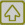Analytical Techniques in Aquaculture Research Main menu < Analysis of Feed & Feed Ingredients < Proximate Analysis < Nitrogen Free Extract

#### Proximate analysis: Nitrogen Free Extract (NFE)

 Principle and ScopeA very inaccurate name indeed. This fraction has nothing to do with nitrogen and it's not an extract either. NFE supposedly represents the soluble carbohydrate of the feed, such as starch and sugar. Crude fiber represents insoluble carbohydrate.

 Calculation% NFE = % DM - (% EE + % CP + % ash + % CF)

where: NFE = nitrogen free extract
DM = dry matter
EE = ether extract or crude lipid
CP = crude protein
CF = crude fiber

 Possible errorsThis is the ONLY component in the Proximate Analysis which is not determined ANALYTICALLY but is calculated by difference. Therefore, NFE accumulates all of the errors that existe in other proximate analysis components (CF is the biggest error)